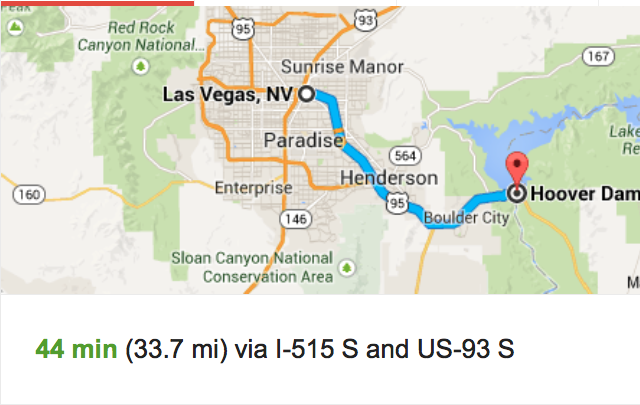## Thursday, January 29, 2015

### Red Zone | Dark Knight Rises and Super Bowl 49Red Zone = 9+5+4+8+6+5+5 = 42

Dark Knight = 4+1+9+2+2+5+9+7+8+2 = 49
Revelation = 9+5+4+5+3+1+2+9+6+5 = 49
Super Bowl 49 is on February 1, 2015
*Dark Knight = 4+1+9+11+11+5+9+7+8+2 = 67
*Revelation = 9+5+22+5+3+1+2+9+6+5 = 67

Did you notice the 'Rogue Wave' sign?  Imagine if the Hoover Dam broke...  I think 'Las Vegas' would be the 'Red Zone'.

• Hines Ward wore 86
• Hines Ward finished his career with 86 Touchdowns
• Deflate Gate = 4+5+6+12+1+20+5+7+1+20+5 = 86
• Las Vegas = 12+1+19+22+5+7+1+19 = 86
• Triangle = 20+18+9+1+14+7+12+5 = 86
• Pyramid = 16+25+18+1+13+9+4 = 86Boulder City = 2+6+3+3+4+5+9+3+9+2+7 = 53

1.Hoover Dam coordinates: 36°0′56″N 114°44′16″W

2.Boom Boom Out Go The Lights = 2+6+6+4+2+6+6+4+6+3+2+7+6+2+8+5+3+9+7+8+2+10 = 114

3.Lewis, where is this phrase being used? Good work.

4.Some coordinates...

5.Heard it on the radio. A classic rock song made famous by Pat Travers.